﻿## 向量、矩阵的数乘及点积

 数加 数乘/积 互相加 互相乘/点积 数学向量 标量运算即为向量和数字间的运算。向量与数的运算就是向量内每一个元素与这一个数进行相应的运算。如下图的一个标量运算：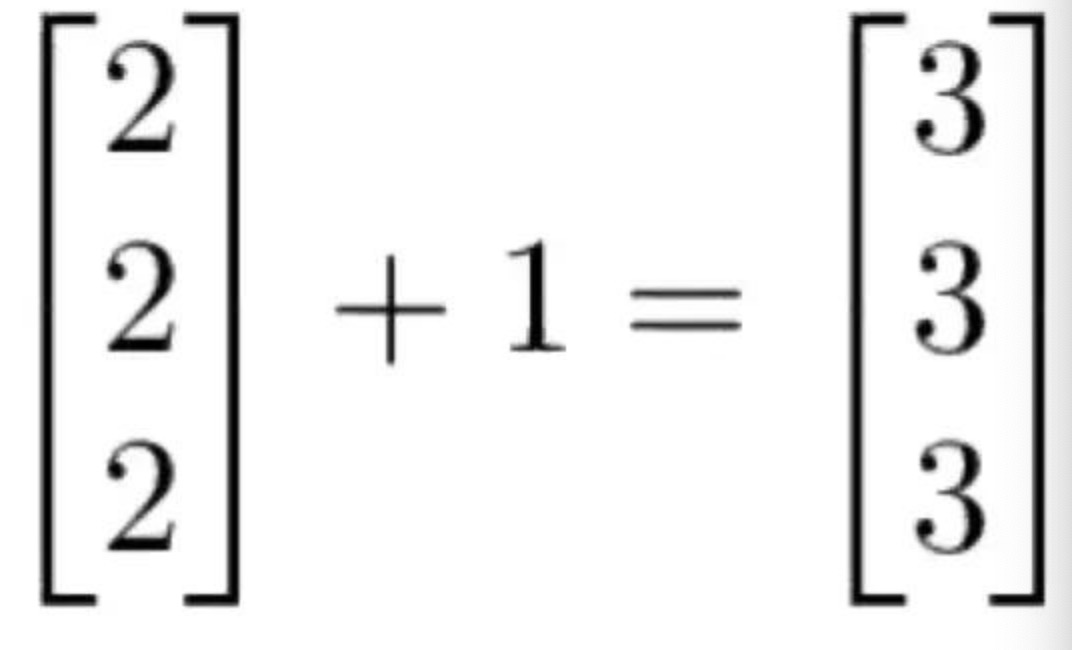一个数乘以一个向量等于这个数同向量中的每个元素相乘 2   [1 2 3] = [2 4 6] 2个向量对应位置的元素相加 [1 2 3] + [1 2 3] = [2 4 6] 一个行向量乘以一个列向量,是两个向量对位相乘再相加,结果是一个实数. |1 2 3 | *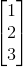= 1*1 + 2*2 + 3*3 = 14 数学矩阵 矩阵的标量运算矩阵的标量运算和向量的标量运算是一样的。可以简单地将标量和矩阵中的每一个元素做运算处理（如加、减、乘、除等）。 同向量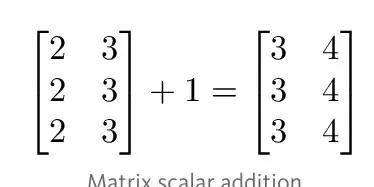同向量 矩阵间的运算为了能进行加减运算，两个矩阵的阶必须相等。然后我们可以对两个矩阵相应的元素进行运算处理。如下图就是两阶方阵的加法。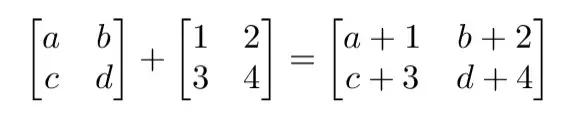m×n 阶矩阵左乘 n×k 阶矩阵的结果是 m×k 阶矩阵 矩阵乘法的步骤和向量点积的过程是相似的，它们都是由对应位置的元素进行乘积并相加而得出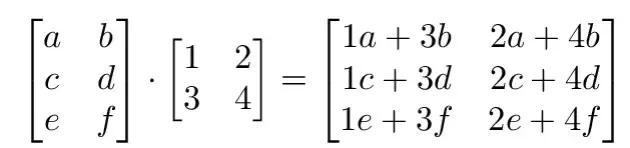python列表 不能操作 重复列表 ['a','b']  * 2 结果为 ['a', 'b', 'a', 'b'] 列表拼接 ['a','b'] + [1, 2] 结果为 ['a',  'b', 1, 2] 不能操作 np.array 同数学矩阵 同数学矩阵 同数学矩阵 见以下例子，np.multiply()和np.dot() np.matrix 同数学矩阵 同数学矩阵 同数学矩阵 见以下例子，np.multiply()和np.dot()

### 1 np.multiply()函数

```A = np.array([[0,1],[2,3]])
B = np.array([[1,2],[3,4]])
print(np.multiply(A, B))       # 数组对应位置元素相乘

array([[0,  2],
[6, 12]])```

### 2 np.dot() 函数

```A = np.array([[0,1],[2,3]])
B = np.array([[1,2],[3,4]])
print(np.dot(A, B))   # 执行矩阵乘法运算

array([[3,  4],
[11, 16]])
```